F4 Next: The Magic Square Up: Exceptional Lie Algebras Previous: G2

## 4.2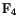The second smallest of the exceptional Lie groups is the 52-dimensional group. The geometric meaning of this group became clear in a number of nearly simultaneous papers by various mathematicians. In 1949, Jordan constructed the octonionic projective plane using projections in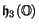. One year later, Armand Borel  noted thatis the isometry group of a 16-dimensional projective plane. In fact, this plane is none other than than. Also in 1950, Claude Chevalley and Richard Schafer  showed thatis the automorphism group of. In 1951, Freudenthal  embarked upon a long series of papers in which he described not onlybut also the other exceptional Lie groups using octonionic projective geometry. To survey these developments, one still cannot do better than to read his classic 1964 paper on Lie groups and the foundations of geometry .

Let us take Chevalley and Schafer's result as the definition of: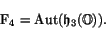Its Lie algebra is thus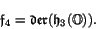As we saw in Section 3.4, points ofcorrespond to trace-1 projections in the exceptional Jordan algebra. It follows thatacts as transformations of. In fact, we can equipwith a Riemannian metric for whichis the isometry group. To get a sense of how this works, let us describeas a quotient space of.

In Section 3.4 we saw that the exceptional Jordan algebra can be built using natural operations on the scalar, vector and spinor representations of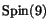. This implies thatis a subgroup of. Equation (3.4) makes it clear thatis precisely the subgroup fixing the element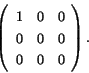Since this element is a trace-one projection, it corresponds to a point of. We have already seen thatacts transitively on. It follows that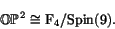This fact has various nice spinoffs. First, it gives an easy way to compute the dimension of:Second, sinceis compact, we can take any Riemannian metric onand average it with respect to the action of this group. The isometry group of the resulting metric will automatically includeas a subgroup. With more work , one can show that actually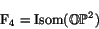and thus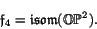Equation (4.2) also implies that the tangent space of our chosen point inis isomorphic to. But we already know that this tangent space is just, or in other words, the spinor representation of. We thus have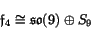as vector spaces, whereis a Lie subalgebra. The bracket in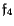is built from the bracket in, the action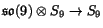, and the map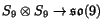obtained by dualizing this action. We can also rewrite this description ofin terms of the octonions, as follows: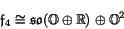This last formula suggests that we decomposefurther using the splitting of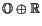intoand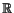. It is easily seen by looking at matrices that for allwe have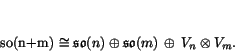Moreover, when we restrict the representation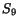to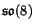, it splits as a direct sum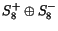. Using these facts and equation (4.2), we seeThis formula emphasizes the close relation betweenand triality: the Lie bracket inis completely built out of maps involvingand its three 8-dimensional irreducible representations! We can rewrite this in a way that brings out the role of the octonions:While elegant, none of these descriptions ofgives a convenient picture of all the derivations of the exceptional Jordan algebra. In fact, there is a nice picture of this sort for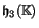whenever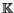is a normed division algebra. One way to get a derivation of the Jordan algebrais to take a derivation ofand let it act on each entry of the matrices in. Another way uses elements ofGiven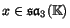, there is a derivation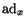ofgiven by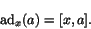In fact , every derivation ofcan be uniquely expressed as a linear combination of derivations of these two sorts, so we have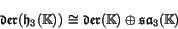as vector spaces. In the case of the octonions, this decomposition says that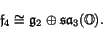In equation (4.2), the subspace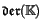is always a Lie subalgebra, butis not unlessis commutative and associative — in which casevanishes. Nonetheless, there is a formula for the brackets inwhich applies in every case . Given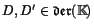and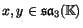, we have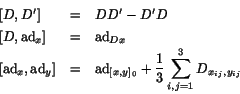where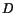acts oncomponentwise,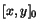is the trace-free part of the commutator, and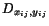is the derivation ofdefined using equation (4.1).

Summarizing these different descriptions of, we have:

Theorem 5.   The compact real form ofis given by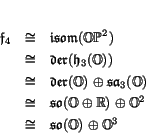where in each case the Lie bracket is built from natural bilinear operations on the summands.

Next: The Magic Square Up: Exceptional Lie Algebras Previous: G2